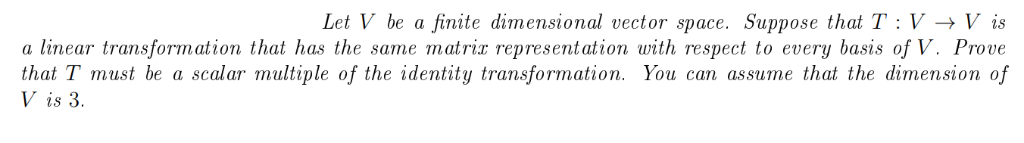# Solved: Let V Be A Finite Dimensional Vector Space. Suppose That T : V -> V Is A Linear Transformation That Has The Same ?atriz Represe?ntation With Respect To Every Basis Of V. Prove That T Must Be A

By |Let V be a finite dimensional vector space. Suppose that T : V -> V is a linear transformation that has the same ?atriz represe?ntation with respect to every basis of V. Prove that T must be a scalar multiple of the identity transformation. You can assume that the dimension of V is 3